# How to Show or Hide Chart Axis in Excel

This post will guide you how to show or hide chart Axis in your current worksheet in Excel 2013/2016. How do I show or hide Axes or 3-D chart Axes, Horizontal Axis, Vertical Axis or secondary Vertical Axis in your chart in Excel.

Normally, Once you create a newly chart based on your data in your worksheet, the vertical Axis and the horizontal Axis are displayed automatically in Excel. But for some cased, it is not necessary to display all or any of the axes for your specified chart. You can try to hide chart axis in Excel.

## What is Axis in Excel

Each axis is a line on a chart that together define its structure and which the data plotted on the graph is measured against. The Y axis is the vertical access, in other words the one that goes up and down. The X access is horizontal and so it goes across a chart. The Y axis is sometimes known as the value axis. Having an axis is common on scatter diagrams or X-Y Charts, bar charts and others. You do not have one on a pie chart. Most of Chart types have two axes: vertical axis and horizontal axis.

In addition to vertical and horizontal axes, there is another axis called 3-D chart Axes, and also called the secondary vertical axis or depth axis, and it will plot data along the third dimension of a chart.

The secondary vertical axis shows the values for the associated data series, and it works well in a chart that show a combination of column and line charts.

## Hide and Display Chart Axes

If you want to hide one or more axes in your excel chart in your current worksheet, and just do the following steps:

Step1: select a blank area of your chart, then the Chart Tools will be displayed on the right side of the chart.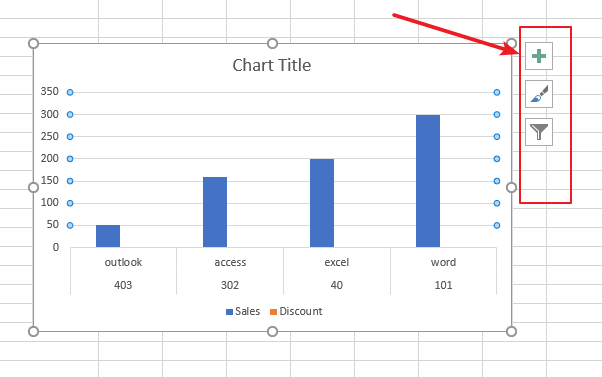Step2: select Chart Elements button on the Chart Tools, and click the plus sign(+), and the Chart Elements menu will appear.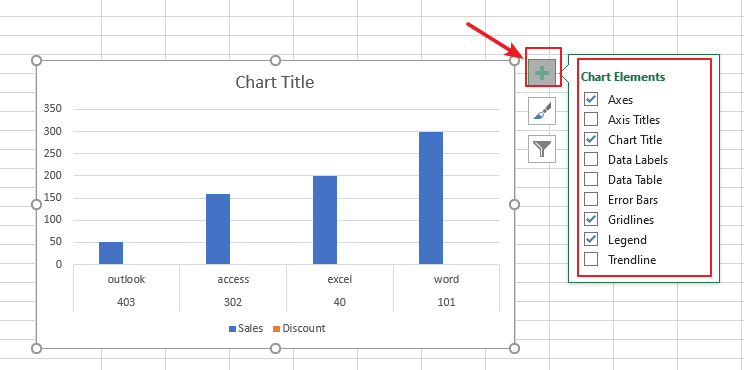Step3: if you want to hide all chart axes, just uncheck the Axes check box.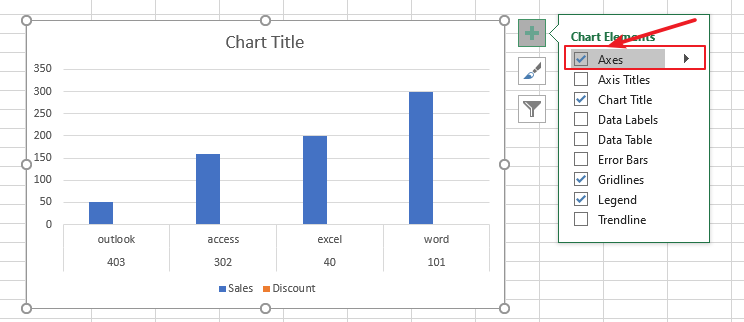Step4: if you want to hide primary or secondary axis or more axes, and you need to click the right arrow to display a list of axes that can be hidden or displayed on the chart as you need. You just need to uncheck the check box for the axes that you want to hide.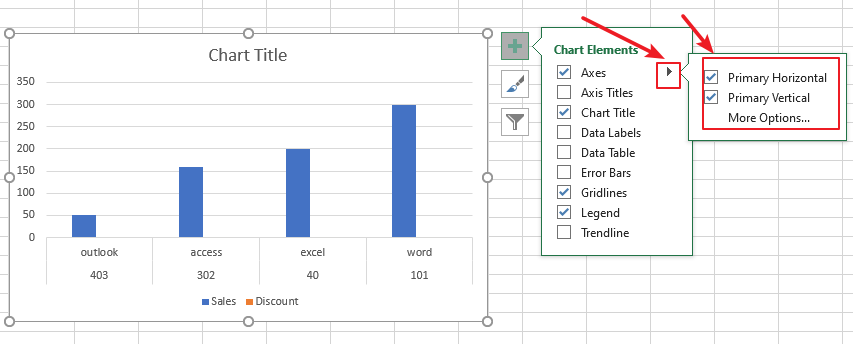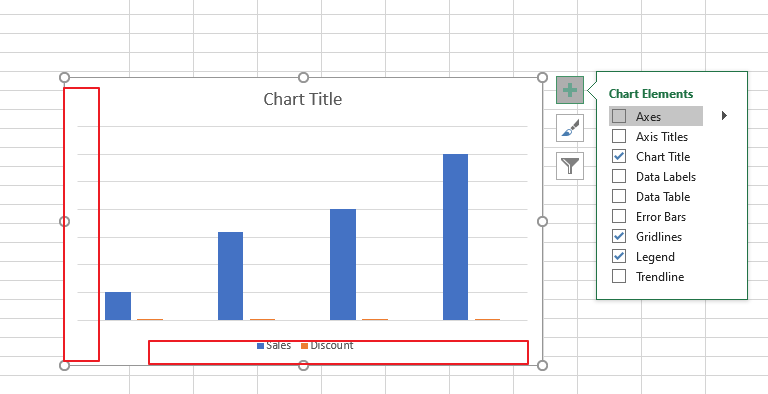Step5: To show one or more chart axes or one specified axis, and you just need to follow the above steps to check the check box for the axes that you want to display.

Related Posts

Cap percentage values between 0 and 100

This article will talk about how to cap the percentage values between 0% and 100% in Microsoft Excel Spreadsheet or Google Sheets. If you are a newbie on Excel or google Sheets, you may be able to do this by ...

Calculate Cap Percentages to Specific Value

This article will talk about how to limit the cap percentage of a given amount to a given value in Microsoft Excel Spreadsheet or Google Sheets. If you are a newbie on Excel or google Sheets, you may be able ...

Calculate Win Loss Tie

Suppose you got a task to calculate the win, loss, and tie totals; what would you do? If you are new to Ms Excel and don't have enough experience with it, then you might do this task manually but let ...

Calculate Years Between Dates In Ms Excel

If you are an avid Ms Excel user, then you might have come across a task in which you needed to calculate the years between the dates; you might take it easy and do this task manually, which is also ...

Calculate Number of Hours between Two Times

Calculating the difference between two times might be a valuable statistic for subsequent computations or averages, whether you're producing a time sheet for staff or recording personal exercises. While Excel has a plethora of complex functions, including date and time ...

Calculate Loan Interest in Given Year

When you borrow money, you are supposed to repay it gradually. Lenders, on the other hand, want to be compensated for their services and the risk they incur by lending you money. That is, you will not just repay the ...

Calculate Interest Rate for Loan

The interest rate is the fee charged by a lender to a borrower and is expressed as a percentage of the principal—the lent amount. The interest rate on a loan is often expressed as an annual percentage rate, abbreviated as ...

Calculate Interest for Given Period

Using the IPMT function in Excel, we can compute the interest payment on any loan. This step-by-step tutorial will guide Excel users of all skill levels through the process to calculate interest for given period. Finally, the formula: =IPMT(B3/12,1,B5,-B2) The ...

How To Use Excel GCD Function

This post will guide you how to use Excel GCD function with syntax and examples in Microsoft excel. Description The Excel GCD function Returns the greatest common divisor of two or more integers. So you can use the GCD function ...

Calculate A Ratio From Two Numbers In Excel

In elementary mathematics, a ratio is a connection or comparison between two or more integers. For example, ratios are often expressed as ":" to demonstrate the relationship between two numbers. You would think that manually calculating a ratio from two ...

Sidebar